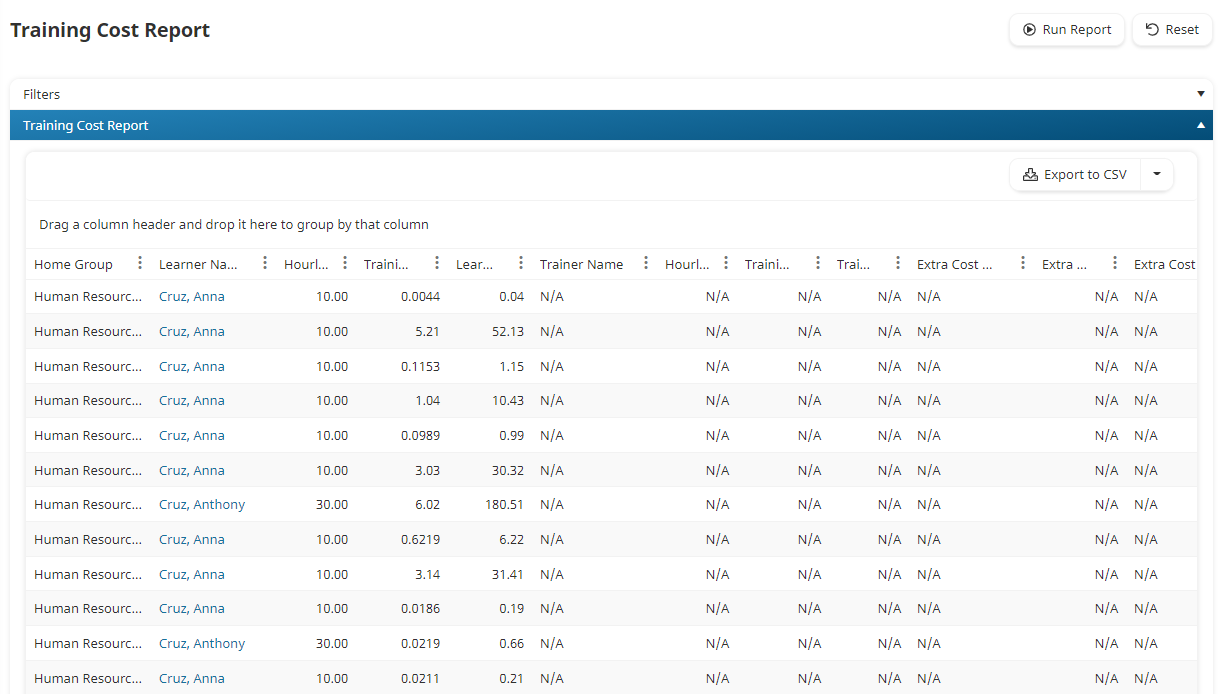Training Cost Report
• Dark
Light

# Training Cost Report

• Dark
Light

Article Summary

This feature is a special component of SmarterU that is not included with the basic system. Please contact our Success Desk to learn how you can upgrade your account to include it.

The Training Cost Report enables you to calculate your direct training costs. The report considers factors such as:Because a learner or instructor's wage may change over time, the report considers the date when a course was completed or when an action was accepted to determine which wage to use in its calculation. For example, suppose a user's wage history is as follows:

Effective DateWage

February 12, 2021

\$12.00

April 30, 2021

\$15.00

July 2, 2021

\$17.50

If the user completed a course on February 1, 2021, the training cost would be \$0.00 since there was no wage entered for the user on that date.

If the user spent 10 hours on an online course and completed it on March 1, 2021, the total cost would be \$120. This was calculated by multiplying the number of hours the user spent on the course (i.e., 10) by the user's wage on March 1, 2021 (i.e., \$12).

If the user spent 2 hours completing an action and the action was accepted on August 14, 2021, the total cost would be \$35. This was calculated by multiplying the number of hours the user took to complete the action (i.e., 2) by the user's wage on the date the action was accepted (i.e., \$17.50).

In addition to the user's wage, the instructor's wage and additional costs are also considered. For example, suppose the user completed an 8-hour instructor-led course on May 4, 2021. Suppose that the instructor's wage at that time is \$20/hour and that there's extra \$100 cost associated with the course. The total cost would be calculated as follows:

 Learner Cost \$15 x 8 = \$120 Instructor Cost \$20 x 8 = \$160 Extra Cost \$100 Total Cost = \$380